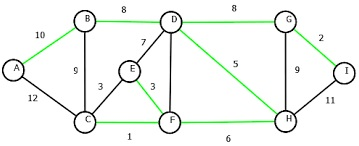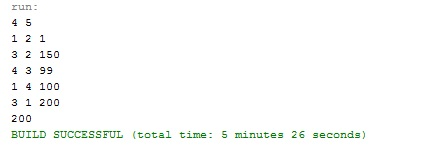# Solution of minimum spanning tree problem in Java using Kruskal's Algorithm

Learn: what is Kruskal’s algorithm and how it should be implemented to find the solution of minimum spanning tree? In this article, we will implement the solution of this problem using kruskal’s algorithm in Java.
Submitted by Anamika Gupta, on June 04, 2018

In Electronic Circuit we often required less wiring to connect pins together. We can model this wiring problem with a connected, undirected graph G=(V, E), where V is the set of pins, E is the set of possible interconnections between pair of pins, and for each edge we have a weight w(u,v) specifying the cost (amount of wire needed) to connect u and v. We then wish to find an acyclic subset T that connects all of the vertices and whose total weight w(T)= sum of all the weights in T is minimized . Since T is acyclic and connects all of the vertices, it must form a tree, which we call a spanning tree since it spans the graph G. We call this problem minimum spanning tree problem.MST Green color edges are the selected edges for MST.

There are two algorithm to solve this problem: Kruskal's Algorithm and Prim's Algorithm. Each of them run in O(E lg V )

Here we are discussing Kruskal's Algorithm...

## Kruskal's Algorithm

This Algorithm first makes the forest of each vertex and then sorts the edges according to their weights, and in each step, it adds the minimum weight edge in the tree that connects two distinct vertexes that do not belong to the same tree in the forest.

It uses a disjoint set data structure to maintain several disjoint sets of elements. Each set contains the vertices in one tree of the current forest.

Example: Here we are finding the cost of MST.

Program:

```import java.io.*;
import java.util.*;
import java.text.*;
import java.math.*;
import java.util.regex.*;

public class MST{

static class set{
int parent,rank;
}

//find set which represents vertex i
static int find(set subsets[],int i ){
if(subsets[i].parent==i)
return i;
return find(subsets,subsets[i].parent);
}

//function for adding  edges whose vertex belongs
//to the different tree ie. UNION
static void UNION(set subsets[],int x,int y){
int xroot=find(subsets,x);
int yroot=find(subsets,y);

if(subsets[xroot].rank>subsets[yroot].rank)
subsets[yroot].parent=xroot;
else if(subsets[xroot].rank<subsets[yroot].rank)
subsets[xroot].parent=yroot;
else{
subsets[yroot].parent=xroot;
subsets[xroot].rank++;
}
}

static int mst(int n, Integer[][] edges) {
set subsets[]=new set[n];

//Create forest of vrtices that is separate tree for each vertex
for(int i=0;i<n;i++)
{
subsets[i]=new set();
subsets[i].parent=i;  // Each vertex is its own parent
subsets[i].rank=0;   //Having no child
}

int e=0,i=0,count=0;
//Create graph having exactly vertex-1 ie. n-1 edges
while(e<n-1){
//find set from which current vertex belongs
int x=find(subsets,edges[i]-1);
//find set from which current vertex belongs
int y=find(subsets,edges[i]-1);

if(x!=y){
count+=edges[i];
e++;
// union the two vertex in the same tree
//if they belong to the different set
UNION(subsets,x,y);
}
i++;
}
return count;
}

public static void main(String[] args)
{
Scanner in = new Scanner(System.in);
int n = in.nextInt();   //number of nodes
int m = in.nextInt();  //number of edges
Integer [][]edges = new Integer[m];
for(int edges_i = 0; edges_i < m; edges_i++){
for(int edges_j = 0; edges_j < 3; edges_j++){
edges[edges_i][edges_j] = in.nextInt();
}
}

//Sort edges two dimensional array according to
//its third column i.e. weight
Arrays.sort(edges,new Comparator<Integer[]>(){
@Override
public int compare(Integer[] i1,Integer[] i2){
//Comparing third column having index 2
return i1.compareTo(i2);
}
});

int result=mst(n,edges);
System.out.println(result);
in.close();
}
}
```

OutputWhat's New

Top Interview Coding Problems/Challenges!

Languages: » C » C++ » C++ STL » Java » Data Structure » C#.Net » Android » Kotlin » SQL
Web Technologies: » PHP » Python » JavaScript » CSS » Ajax » Node.js » Web programming/HTML
Solved programs: » C » C++ » DS » Java » C#
Aptitude que. & ans.: » C » C++ » Java » DBMS
Interview que. & ans.: » C » Embedded C » Java » SEO » HR
CS Subjects: » CS Basics » O.S. » Networks » DBMS » Embedded Systems » Cloud Computing
» Machine learning » CS Organizations » Linux » DOS
More: » Articles » Puzzles » News/Updates